# Disjoint sets

The disjoint sets problem is that of efficiently maintaining a collection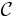$\mathcal{C}$ of disjoint subsets of some universe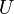$U$. Specifically, we assume that initially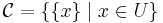$\mathcal{C} = \{\{x\} \mid x \in U\}$, that is, each element of the universe is contained in a singleton. Then, we wish to efficiently support an intermixed sequence of two operations:

1. Find the set that contains a given element$x$. By this we mean that we imagine we have assigned some unique identifier to each set in our collection, so that if we execute two find operations in succession on two elements that are located in the same set, then the same value is returned, whereas different values are returned if they are in different sets.
2. Unite two given sets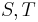$S, T$ in the collection, that is, remove$S$ and$T$ from$\mathcal{C}$, and add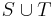$S \cup T$ to$\mathcal{C}$.

For this reason, the disjoint sets problem is often called the union-find problem.

A configuration of disjoint sets is a model of an equivalence relation, with each set an equivalence class; two elements are equivalent if they are in the same set. Often, when discussing an equivalence relation, we will choose one element in each equivalence class to be the representative of that class (and we say that such an element is in canonical form). Then the find operation can be understood as determining the representative of the set in which the query element is located. When we unite two sets, we either use the representative of one of the two sets as the representative of the new, united set, or we pick a new element altogether to be the representative.

The graph-theoretic application of disjoint sets to connectivity may illustrate the problem more clearly. We let the elements of our universe be nodes in an undirected graph, and we say two nodes belong to the same equivalence class if they are in the same connected component. Then, executing Find on a node allows us to identify which connected component it is in; so that if we have two nodes and we execute Find on each one, and the results are the same, then these two nodes are in the same connected component and are mutually reachable. If we add an edge between these two nodes, and they are not already in the same connected component, then we are uniting their respective connected components into one; this corresponds to the Unite operation.

## A first idea

Suppose the elements of the universe are numbered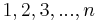$1, 2, 3, ..., n$. Then the first idea we might have is to maintain an array of size$n$ in which the$n$th element is the ID of the representative of the set containing the element numbered$n$. So initially every element is located in its own set, and since this set contains only the element alone, it must be its own representative. Then the array has 1 at index 1, 2 at index 2, and so on. When we execute the find operation on an element, we just look up its representative in the table, which takes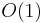$O(1)$ time. When we have to unite two sets, however, we have to pick one of these two sets and change the representative of every element in this set to the representative of the other set. That is, we potentially have to change$O(n)$ elements of the array in order to reflect the union. This solution is satisfactory when there are very few union operations executed, but it leaves a lot to be desired for applications in which there are more unions than finds.

### Example

The universe contains five objects: red, yellow, green, blue, and violet balls. Here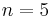$n=5$ and we shall arbitrarily assign the number 1 to refer to the red ball, 2 to refer to the yellow ball, and so on. Initially our collection is {{red},{yellow},{green},{blue},{violet}}, and the array holds the values [1,2,3,4,5], as each ball is its own representative. Now suppose we unite the yellow and violet sets, obtaining {{red},{yellow,violet},{green},{blue}}. To indicate that yellow is in the same set as violet now, we will set violet's representative to 2, giving [1,2,3,4,2] as our array. Now suppose we unite green and blue. Then our collection becomes {{red},{yellow,violet},{green,blue}} and we set blue's representative to 3 to indicate that it is now in the same set as blue, giving [1,2,3,3,2]. If we now execute find on green, we see that the representative 3 is the green ball, and the same goes when we execute find on blue because green and blue are in the same set, but if we execute find on yellow, we get 2, the yellow, ball, which is different, because yellow is not in the same set as green and blue. Finally, suppose we join the {yellow,violet} and {green,blue} sets. We may do this by setting the representatives of both green and blue to 2 (which is the ID of the {yellow,violet}) group, giving [1,2,2,2,2], reflecting that now red is in one group, and the other balls are in the other group. Note that when we unite two groups, it doesn't matter which group we choose to "keep" its representative and which one's members are forced to change.

## Faster union

The implementation described above supports$O(1)$ find, but requires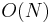$O(N)$ time for union'. What if we focus on trying to improving the speed of the union operation, possibly sacrificing efficiency in find in the process?

We shall do this by maintaining each set as a rooted tree, so that the collection of sets at any given time is a forest (which is sometimes referred to as a disjoint sets forest in discussion of the disjoint sets problem). The representative of any set is at the root of that set's tree. Initially each element is in a tree with only one vertex, and hence is the root.

We maintain an array p such that p[i] gives the ID of the element corresponding to the parent (in the tree) of the node corresponding to the element with ID i, or i itself if this is the root of its tree. Initially, then, p[i] equals i for each i. To carry out the find operation on an element with ID x, we consider the sequence x, p[x], p[p[x]], ..., until an element in this sequence is the same as the one preceding it, at which point we know we have reached the root. Since no cycles can occur, this step runs in$O(n)$ time.

The motivation for this representation is that if we want to join together the sets with representatives with IDs x and y, then all we have to do is set p[x] = y (or vice versa); then, executing find from any vertex initially in x's tree causes the loop to stop not at x but now at y, which is therefore the new root for what was originally two separate trees. So, now, to execute the union operation on the trees rooted at x and y takes only$O(1)$ time.

(There is, however, a catch: if we are given elements from the two sets we wish to unite, but they are not the representatives, then we have to execute find on each of the two given elements first, so this implementation will actually give$O(N)$ time for both operations.)

Now, notice that the find operation, in this implementation, although$O(N)$, is still somewhat of an improvement over the$O(N)$ union of the previous implementation. The$O(N)$ union uses time linear in the size of one of the sets, because it has to reconfigure every element in the set. But the$O(N)$ find takes time linear in the depth of the node queried in its subtree. On the average case, the trees formed by this technique are fairly well-balanced, and it performs much better than the previous one. In the worst case, however, when the trees degenerate into tall paths, the behavior is no better than it was before.

## Union by rank

We see that the algorithm the preceding section performs well on the average case because the trees are fairly well-balanced, so that find occurs relatively quickly and so does union ($O(1)$ time plus the time taken to find the roots of the two nodes given). Is there a way to ensure that the trees are indeed well-balanced?

There is a simple technique that does exactly this, and is called union by rank. This algorithm works in the same way as the preceding one, except that in each root node we keep a count of the size of its tree (initially 1 for each node), and when we join two root nodes, we always make the root of the larger tree the parent of the root of the smaller tree (updating the count field accordingly), breaking ties arbitrarily.

Lemma: With union by rank, at any given time, any tree of height$h$ will contain at least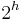$2^h$ nodes.

Proof: By induction on the number of union operations performed so far (finds have no effect on the structure of the forest). When this is zero, we have the initial configuration, in which each tree has height 0 and size 1, so the hypothesis is true. Now, suppose that the current configuration satisfies the inductive hypothesis and that we join together two trees rooted at nodes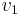$v_1$ and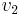$v_2$, with heights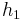$h_1$ and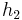$h_2$ and sizes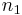$n_1$ and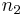$n_2$, respectively. Suppose without loss of generality that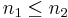$n_1 \leq n_2$, so that we make$v_2$ the parent of$v_1$. Then, there are two cases:

1.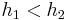$h_1 < h_2$. In this case the new tree still has height$h_2$ and size$n_1 + n_2 \geq 2^{h_1} + 2^{h_2} \geq 2^{h_2}$, and the inductive hypothesis continues to hold.
2.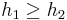$h_1 \geq h_2$. Here the new tree has height$h_1 + 1$ and size$n_1 + n_2 \geq n_1 + n_1 \geq 2^{h_1} + 2^{h_1} = 2^{h_1+1}$, and the inductive hypothesis continues to hold.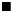$_{\blacksquare}$

Since no tree can ever have more than$n$ nodes, it follows that no tree will ever have height greater than$\lfloor \lg n \rfloor$. And find and union both take time proportional to the height of the trees in which they are executed, so this gives$O(\log N)$ for both operations. This is good enough to give the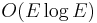$O(E \log E)$ upper bound for the running time of Kruskal's algorithm.

## Path compression

A technique that further improves the speed is path compression. This technique is based on the observation that the more we flatten the trees in the disjoint-set forest, the better the speed becomes (conversely, when the trees are very tall, which is what we found we could avoid with union by rank, the runtime is very poor). Union by rank already guaranteed that the trees are relatively flat; in a sense, they are "at least binary" (the more children the nodes have, the better).

Notice that we can take any node in a tree and change its parent to any other node in that tree without interfering with the correctness of the algorithm, as long as we do not introduce any cycles. In particular, we can take any non-root node in a tree and set its parent to the root, so that future find operations (and, consequently, union operations) involving that node will be much faster. The modification to our basic algorithm is simple: during any find operation, once we eventually find the root of the tree, we change the parent pointer of every node encountered along the path so that it now points to the root node. Asymptotically, this does not increase the amount of work we have to do during a find operation --- it is still linear in the depth of the query node. But as a consequence of the path compression, when used in conjunction with union by rank, the trees become almost completely flat --- so flat, in fact, that the running time for both the find and union operations becomes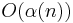$O(\alpha(n))$, which is asymptotically optimal. (Here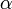$\alpha$ denotes the inverse of the Ackermann function$A$. Because the Ackermann function is extremely fast-growing, the inverse$\alpha$ is extremely slow-growing.)